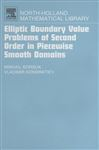# Elliptic Boundary Value Problems of Second Order in Piecewise Smooth DomainsThe book contains a systematic treatment of the qualitative theory of elliptic boundary value problems for linear and quasilinear second order equations in non-smooth domains. The authors concentrate on the following fundamental results: sharp estimates for strong and weak solutions, solvability of the boundary value problems, regularity assertions for solutions near singular points.

Key features:

* New the Hardy – Friedrichs – Wirtinger type inequalities as well as new integral inequalities related to the Cauchy problem for a differential equation.
* Precise exponents of the solution decreasing rate near boundary singular points and best possible conditions for this.
* The question about the influence of the coefficients smoothness on the regularity of solutions.
* New existence theorems for the Dirichlet problem for linear and quasilinear equations in domains with conical points.
* The precise power modulus of continuity at singular boundary point for solutions of the Dirichlet, mixed and the Robin problems.
* The behaviour of weak solutions near conical point for the Dirichlet problem for m – Laplacian.
* The behaviour of weak solutions near a boundary edge for the Dirichlet and mixed problem for elliptic quasilinear equations with triple degeneration.

* Precise exponents of the solution decreasing rate near boundary singular points and best possible conditions for this.
* The question about the influence of the coefficients smoothness on the regularity of solutions.
* New existence theorems for the Dirichlet problem for linear and quasilinear equations in domains with conical points.
* The precise power modulus of continuity at singular boundary point for solutions of the Dirichlet, mixed and the Robin problems.
* The behaviour of weak solutions near conical point for the Dirichlet problem for m - Laplacian.
* The behaviour of weak solutions near a boundary edge for the Dirichlet and mixed problem for elliptic quasilinear equations with triple degeneration.

• ;
• ISBN:
• Edition:
• Title:
• Series:
• Author:
• Imprint:

#### Customer Reviews

If you’re using a PC or Mac you can read this ebook online in a web browser, without downloading anything or installing software.

This ebook is available in file types:

This ebook is available in:

#### DRM Free

The publisher has supplied this book in DRM Free form with digital watermarking.

##### Required software

You can read this eBook on any device that supports DRM-free EPUB or DRM-free PDF format.

#### Digital Rights Management (DRM)

The publisher has supplied this book in encrypted form, which means that you need to install free software in order to unlock and read it.

##### Required software

To read this ebook on a mobile device (phone or tablet) you'll need to install one of these free apps: